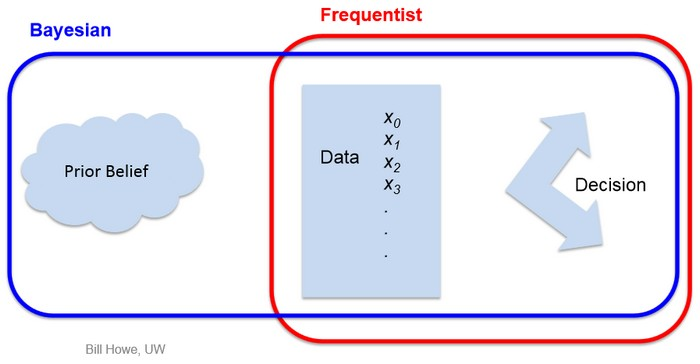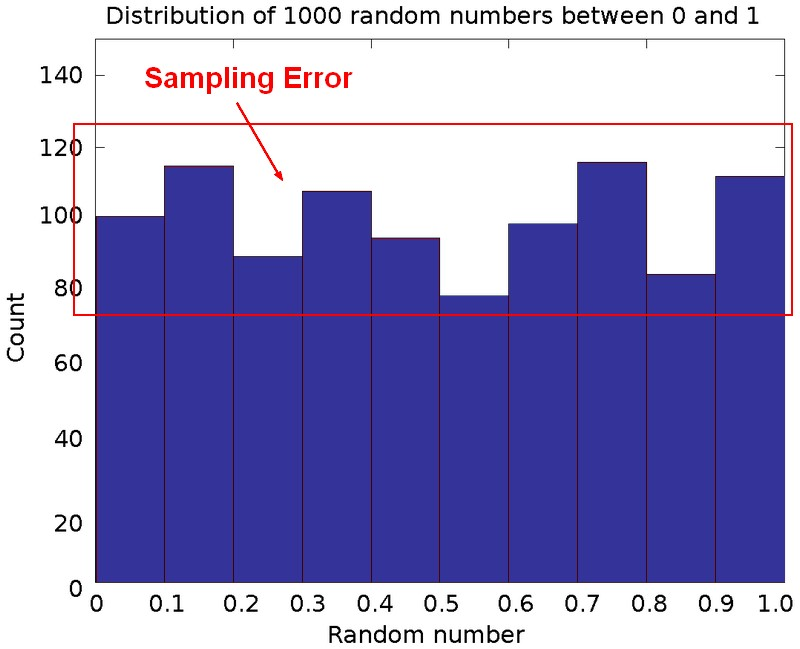# Statistics - Population Parameter

Population parameter must not be confound with a Statistical Parameter

In statistics, all actual numbers about a population are called a parameter. They are never exactly known and are estimated through a statistic as it deals with a sample.

A statistician would not say that the parameter is a specific number, but would most likely report something like the following: “I am fairly confident that the true number is actually between … and ….”

A parameter is:

• a statistical measure or number that summarizes the entire population.
• a numerical measure that describes a characteristic of a population

A parameter describes the population whereas a statistic describes a sample

## Documentation / Reference

Discover More(Mathematics|Statistics) - Statistical Parameter

population parameter A parameter is a numerical characteristic, feature, or measurable factor that help in defining a particular model. Unlike variables, parameters are not listed among the arguments...Machine Learning - (Overfitting|Overtraining|Robust|Generalization) (Underfitting)

A learning algorithm is said to overfit if it is: more accurate in fitting known data (ie training data) (hindsight) but less accurate in predicting new data (ie test data) (foresight) Ie the model...Statistics

is a scientific discipline devoted to the study of data. is the art of extracting information from data. From Data to Information to Knowledge. No learning. lies lies, damned lies, and statistics....Statistics - (Degree|Level) of confidence

Degree of confidence represents the probability that the confidence interval captures the true population parameter. With a degree of confidence of 95%, you have 95% confidence that the true population...Statistics - (Estimation|Approximation)

In statistics, it's always impossible to do experimentation on the entire population. Sample of this population are then used to estimate characteristic of this population (ie the statistics). A sample...Statistics - (Student's) t-test (Mean Comparison)

The t-test is a test that compares means. NHST can be conducted yielding to a p-value Effect Size can be calculated like in multiple regression. Confidence Interval around the mean can also be...Statistics - Confidence Interval

The definition of a confidence interval says that under repeated experiments 95% of the time this confidence interval will contain the true statistic (mean, ...). if we started the whole experiment over...Statistics - Probability (of an event) / Likelihood

The probability of an event is the subjective chance that it will happen. The laws of probability are heavily build with therandom notion. A probability is a non-negative number between 0 and 100%. ...Statistics - Standard Error (SE)

Standard Error is a measure of precision for a statistic (slope, intercept or custom calculations). Standard error is an estimate of amount of sampling error as we typically don’t know the population...Statistics - Statistic

In general, each statistic is an estimate of a parameter, whose value is not known exactly. Every number found using a sample is just an (approximation|estimation) of a parameter (the truth). in descriptive...